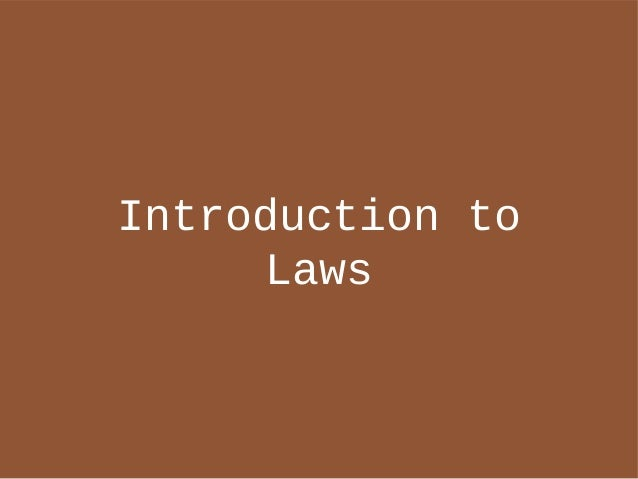Successfully reported this slideshow.
We use your LinkedIn profile and activity data to personalize ads and to show you more relevant ads. You can change your ad preferences anytime.Upcoming SlideShare
×

Introduction to Laws

8,336 views

Published on

Talk given on 2013-01-22 to the BFPG: http://www.bfpg.org/events/87030712/

Video: http://vimeo.com/58236838

Published in: Technology
• Full Name
Comment goes here.

Are you sure you want to Yes No
Your message goes here• Be the first to comment

Introduction to Laws

1. 1. Introduction to Laws
2. 2. About @nkpart Scalaz Functional JavaRuby/Scala/Haskell/Objective-C FunctionalKitMogeneration Kit Various ruby gems
3. 3. Why Laws?They are the footnote in everymonad tutorial.Too hard at the beginning.Programming isn’t even Mathsanyway.
4. 4. Laws are theNew/Old HotnessYou are already programming withLaws.Laws are fundamental tounderstanding most typeclasses.Programming *is* maths.
5. 5. What are Laws Understanding LawsLegal Benefits Breaking Laws
6. 6. What are Laws Common LawsLegal Benefits Breaking Laws
7. 7. (7 + 5) + 3 = 7 + (5 + 3)
8. 8. (a + b) + c = a + (b + c)
9. 9. (a ⊗ b) ⊗ c = a ⊗ (b ⊗ c)
10. 10. What are Laws?Laws are statements made aboutequivalence of expressions.Some Laws come from Maths Abstract Algebra
11. 11. What are Laws?Integer addition obeys theAssociativity LawWherever we use IntegerAddition, we can use theproperties of the law to ouradvantage. 7 + 3 + 5 + 2 = 7 + (3 + 5) + 2For an implementor, the Law is aContract!
12. 12. add :: Int -> Int -> Int
13. 13. What are Laws?Laws are not checked by the typesystemLaws can be broken byimplementationsVerification is usually done byhand Alternatively, QuickCheck.
14. 14. What are Laws?Statements of EquivalenceContracts for the ImplementorLaws are not checked by the typesystemOrigins in Maths. (Programming)
15. 15. What are Laws Common LawsLegal Benefits Breaking Laws
16. 16. Common LawsAbstract Algebra Associative Law Commutative Law IdentityTypeclasses and their Laws Monoid Functor
17. 17. Associative Law(a ⊗ b) ⊗ c = a ⊗ (b ⊗ c) Satisfied by: +, *, concat Uses: Parallelism. String building (refactoring)
18. 18. Associative Law [1,2,3,4,5,6] fold/each/inject1 + (2 + (3 + (4 + (5 + 6)))) apply the law!1 + (2 + ((3 + 4) + (5 + 6))))
19. 19. Commutativity Lawa ⊗ b = b ⊗ a Satisfied by: +, *, but not concat! Uses: Parallelism (again!)
20. 20. Commutative Law1 + (2 + ((3 + 4) + (5 + 6)))) apply the law!1 + (2 + ((5 + 6) + (3 + 4))))
21. 21. Identity Lawa ⊗ Id = a = Id ⊗ a Satisfied by: +/0, */1, concat/ []
22. 22. Typeclasses
23. 23. Monoidclass Monoid a where mappend :: a -> a -> a mempty :: a
24. 24. Monoidmappend satisfies theAssociative Lawmempty is the Identity for themappend operation.
25. 25. class Monoid a where mappend :: a -> a -> a mempty :: a
26. 26. MonoidCODE TIME
27. 27. Functor
28. 28. class Functor f where fmap :: (a -> b) -> f a -> f b
29. 29. Functor Lawsfmap id x = id xfmap (g . h) = (fmap g) . (fmap h)where id a = a id a = a
30. 30. Not a Functorinstance Functor [] where fmap f [] = [] fmap f (x:xs) = (f x):(f x):(fmap f xs)
31. 31. Functor LawsFunctor is a structure with an`fmap` that does not affect thatstructure, just the valuesinside.To modify the structure you needa different typeclass. The lawsprevent it.
32. 32. What are Laws Common LawsLegal Benefits Breaking Laws
33. 33. Legal BenefitsMeaning to Multi-functionTypeclassesGreater understanding ofTypeclassesSubstitution of Expressions
34. 34. Instancing Functor CODE TIME
35. 35. Subsituting ExpressionsSome Haskell tooling can usethisHLintGHC Rewrite Rules
36. 36. HLintCODE TIME
37. 37. Rewrite Rules{-# RULES“map/map” forall f g xs.map f (map g xs) = map (f . g) xs#-}
38. 38. What are Laws Common LawsLegal Benefits Breaking Laws
39. 39. Breaking LawsLawless Typeclasses Pointed (Haskell [deprecated[ and Scalaz [never released]) Zero (Scalaz [never released])Real World Broken Instances Bijection (Twitter), ListT (Haskell Platform), Gen (from QuickCheck)
40. 40. “Lawless Typeclasses”class Zero a where zero :: aclass Pointed f where return :: a -> f a
41. 41. Broken Instances Broken typeclass instances exist Verification is hard. The Gen Monad: CODE TIME
42. 42. Consequences ofIllegal Behaviour
43. 43. Consequences ofIllegal Behaviour Bijection[Int, String]
44. 44. Consequences ofIllegal Behaviour Specific code using a Bijection[Int, String] might be fine. What if I write a function that uses a Bijection[A,B]?
45. 45. What are Laws Understanding LawsLegal Benefits Breaking Laws
46. 46. Laws are GoodLaws have a huge impact on the waywe code (already). Refactoring, Algebraic LawsTaking advantage of laws is apowerful programming technique. Understanding typeclasses, writing new instancesWatch out for Law breakers!
47. 47. Useful ResourcesHaskell Packages- Lens Typeclassopedia- Semigroupoids #scalaz- Pipes #haskell[.au] #bfpgScalaz
48. 48. Thanks!